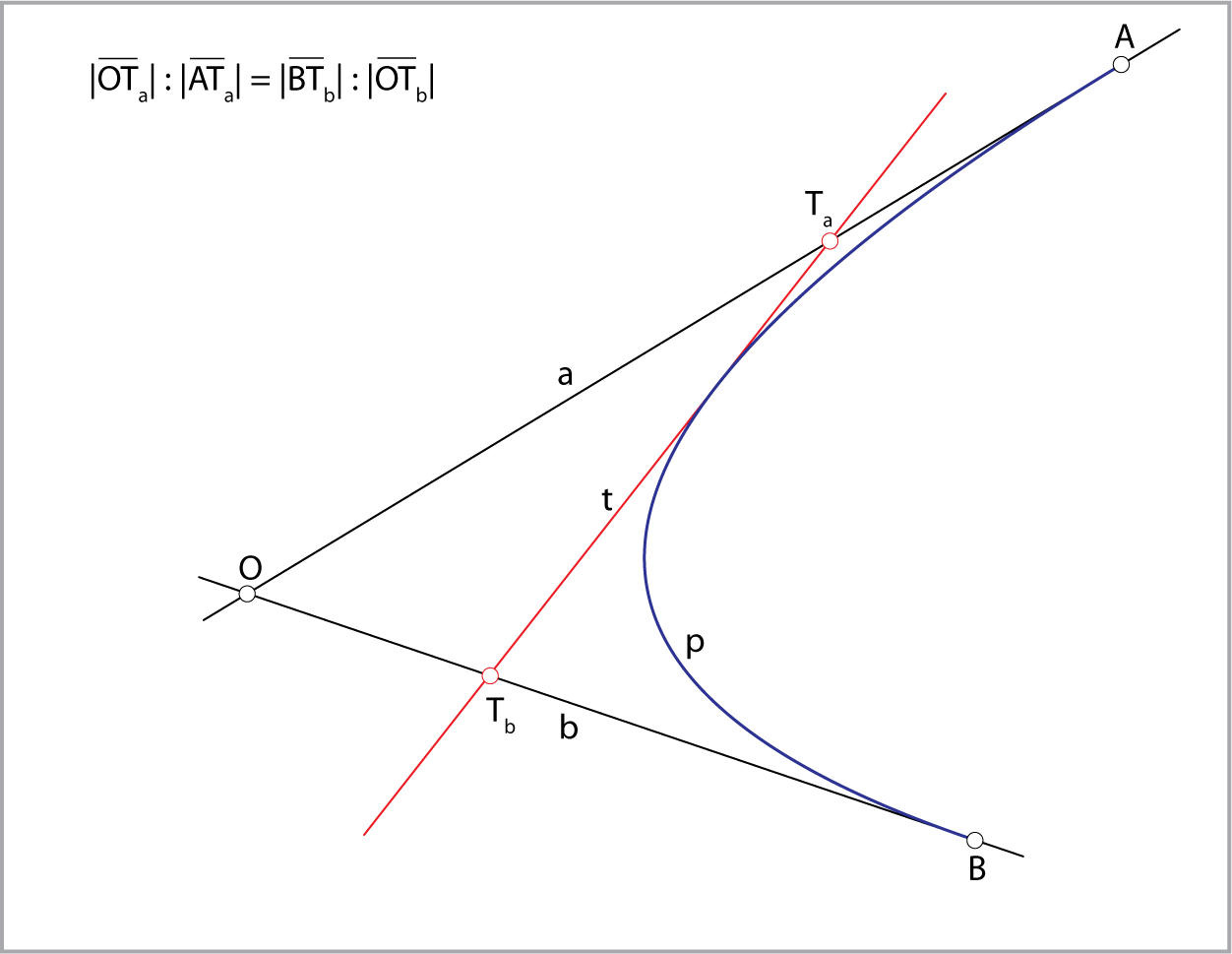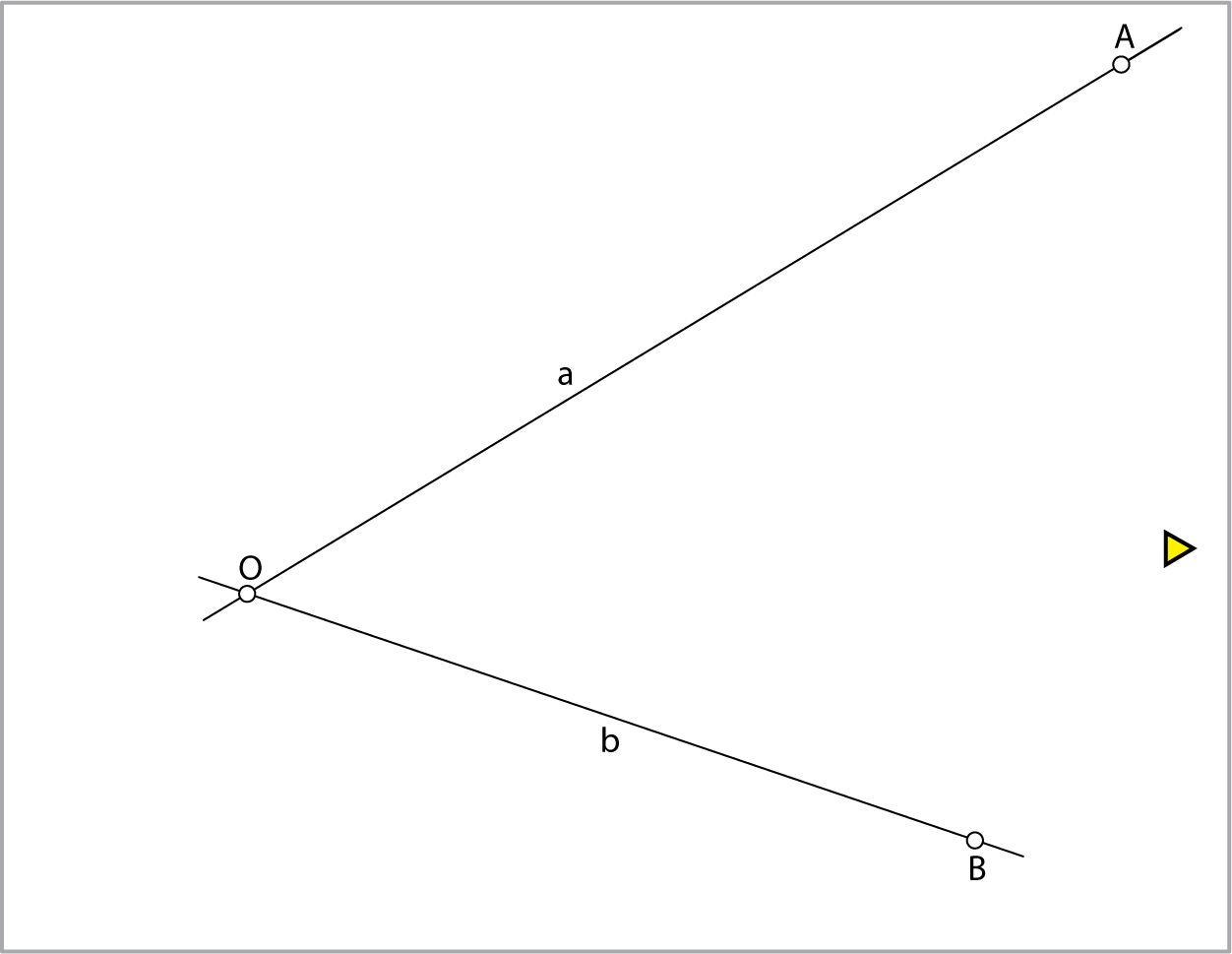### Parabola - envelope of tangent lines

 Let $$a$$ and $$b$$ be the tangent lines of the parabola  $$p$$ with points of tangency $$A$$ and $$B$$ and they intersect at the point $$O$$. Then, for any third tangent line $$t$$ of that parabola, the property depicted in the image below holds.Construction of any number of tangents of the parabola if two tangent lines and their points of tangency are given.created by Sonja Gorjanc, translated by Helena Halas and Iva Kodrnja - DESCRIPTIVE GEOMETRY (lectures)